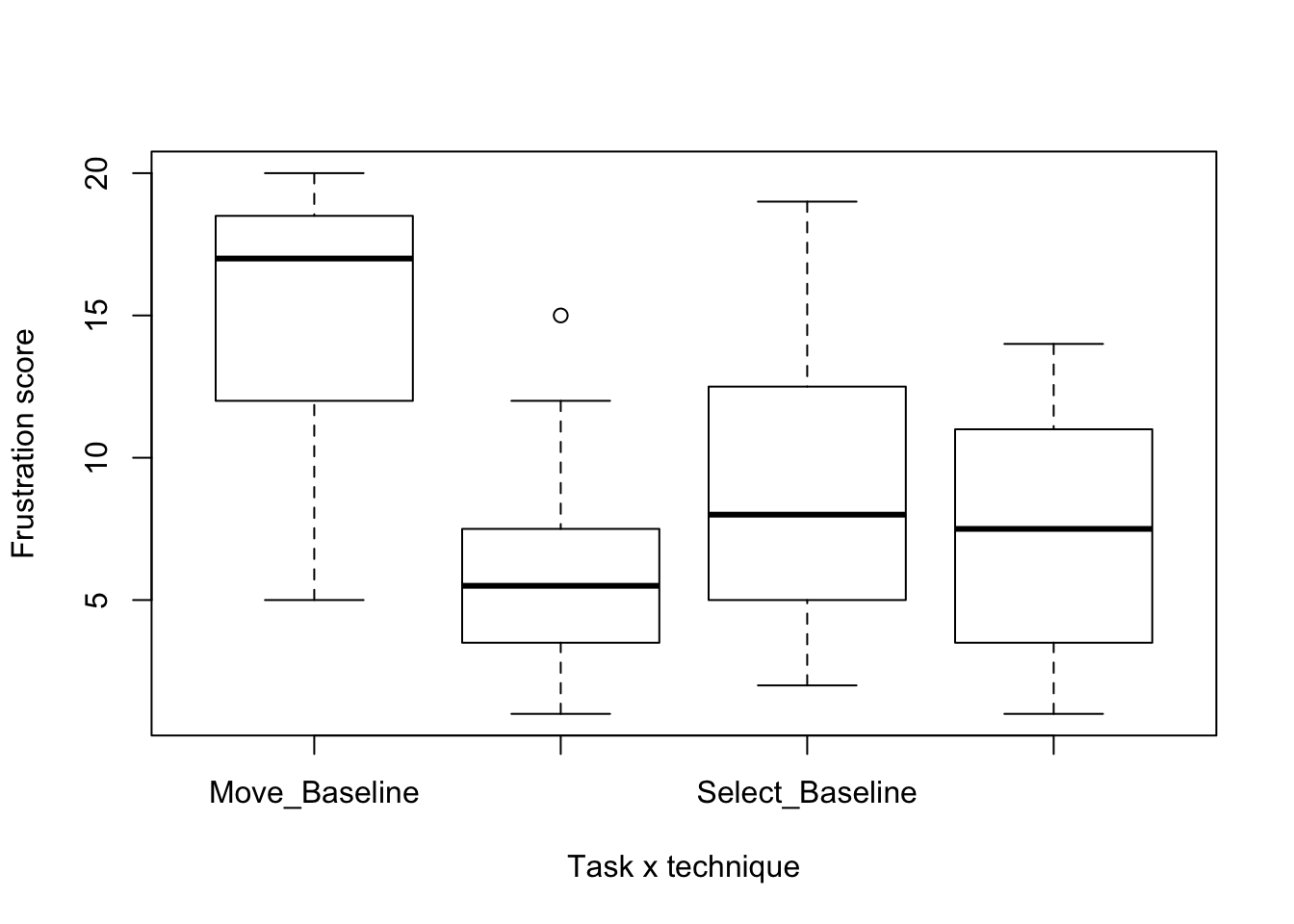data = read.table("ForceEdgeStudy1NasaTLX.csv", header=TRUE, sep=",")

## Filter to keep only frustration

data_filtered = data %>% filter(question == "frustration")

data_aggr = data_filtered %>% unite("task_technique", c("task", "technique"))
mdata = melt(data_aggr, id=c("participant","task_technique","score"))

## Boxplot

boxplot(score~task_technique,data=mdata, xlab="Task x technique", ylab="Frustration score")## Transpose data

data_tr = mdata %>% spread(task_technique,score)
data_tr$participant = NULL data_tr$variable = NULL
data_tr$value = NULL kable(data_tr) Move_Baseline Move_ForceEdge Select_Baseline Select_ForceEdge 20 4 13 5 9 6 11 4 17 1 2 2 13 3 2 3 20 8 6 11 20 12 12 9 17 3 19 8 17 15 15 12 19 4 17 14 18 7 7 13 15 6 2 2 11 5 8 8 7 7 5 11 5 5 5 4 17 1 10 1 14 9 8 7 ## Friedman res = friedman.test(data.matrix(data_tr)) pander(res) Friedman rank sum test: data.matrix(data_tr) Test statistic df P value 21.94 3 6.717e-05 * * * ## Wilcoxon post-hoc analysis res = pairwise.wilcox.test(mdata$score, mdata$task_technique, paired = TRUE, p.adj = "bonf") kable(res$p.value)
Move_Baseline Move_ForceEdge Select_Baseline
Move_ForceEdge 0.0065079 NA NA
Select_Baseline 0.0136319 0.8746468 NA
Select_ForceEdge 0.0049920 1.0000000 1

## Medians

kable(aggregate( score~task_technique, mdata, median ))